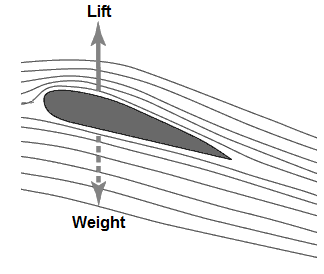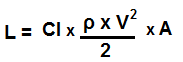﻿ Lift Calculator ﻿# Lift CalculatorL= Cl x q x A

Lift = Lift Coefficient x Dynamic pressure x Area

 Lift Coefficient, Cl: Dynamic pressure, q Pa Area, A m2

0.00 N

This liftcalculator calculates the lift force of an aerodynamic element based on the lift coefficient, Cl, of the element, the dynamic pressure, q, that the element is experiencing, and the area, A, that the element has exposed to generate aerodynamic lift.

The lift force is the upward force that allows an aerodynamic element to go airbone. Being that it is a force, it is measured in unit newtons (N).

The lift coefficient, Cl, is a dimensionless unit that relates the lift force generated to the dynamic pressure times the area of the aerodynamic element. The greater the lift coefficient, the more aerodynamic is the flying element and the greater the lift force that will be generated.

The dynamic pressure, q, is the pressure that is created by an aerodynamic element in motion. Dynamic pressure is equal to one-half times the velocity squared (q= 0.5ρV2). Dynamic pressure is created when the aerodynamic element is in motion. Thus, the greater the velocity of the element, meaning the greater speed at which it is travelling, the greater the dynamic pressure. This is why an airplane is able to generate more lift while it is travelling fast and why it must maintain a certain airspeed in order to be in flight. Otherwise, sufficient lift will not be generated and the airplane will stall in flight and fall out of the air. So the greater the dynamic pressure on the aircraft, the greater the lift force created.

The area, A, is the amount of surface area that can create lift that the aerodynamic element exposes. The greater the area, the more lift that can be created. This is why airplanes have very large wings. The wings have large surface area. This gives a lot of area for an upward force to act on the wings to lift the aircraft. The larger the surface area, the more potential for more lift.

To use this calculator, a user just enters the lift coefficient, Cl, of the aerodynamic element, its dynamic pressure, q, and the area of its wings, and presses the 'Calculate' button. The resultant value will then be automatically computed and shown below.

### Lift Calculator if Dynamic Pressure is Not KnownLift Coefficient, Cl: Density, ρ kg/m3 Velocity, V m/s Area, A m2

0.00 N

This calculator also calculates the lift force if the dynamic pressure is not known upright.

Since dynamic pressure is equal to one-half density times velocity squared, it is substituted into the original formula. This gives us the equation shown above.

To use this calculator, a user just enters the lift coefficient, Cl, the density, ρ, of the fluid the aerodynamic element is moving through, the velocity, v, at which the aerodynamic element is travelling through the fluid, and the area, A, of the aerodyamic elements lift structure. Once the 'Calculate' button is pressed, the resultant value will be computed and displayed.

Related Resources

﻿